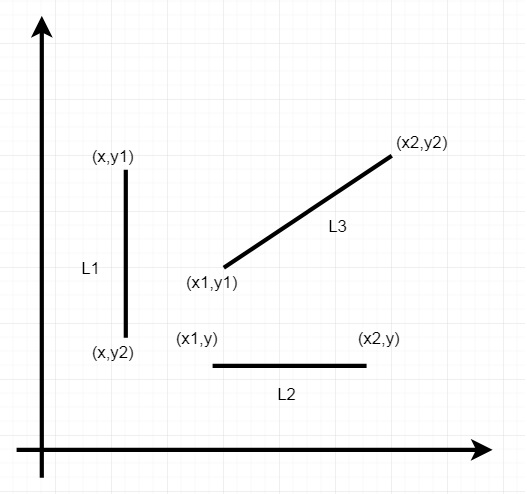# Prolog - Basic Programs

In the following chapter, we are going to discuss basic prolog examples to −

• Find minimum maximum of two numbers

• Find the equivalent resistance of a resistive circuit

• Verify whether a line segment is horizontal, vertical or oblique

## Max and Min of two numbers

Here we will see one Prolog program, that can find the minimum of two numbers and the maximum of two numbers. First, we will create two predicates, find_max(X,Y,Max). This takes X and Y values, and stores the maximum value into the Max. Similarly find_min(X,Y,Min) takes X and Y values, and store the minimum value into the Min variable.

### Program

```find_max(X, Y, X) :- X >= Y, !.
find_max(X, Y, Y) :- X < Y.

find_min(X, Y, X) :- X =< Y, !.
find_min(X, Y, Y) :- X > Y.
```

### Output

```| ?- find_max(100,200,Max).

Max = 200

yes
| ?- find_max(40,10,Max).

Max = 40

yes
| ?- find_min(40,10,Min).

Min = 10

yes
| ?- find_min(100,200,Min).

Min = 100

yes
| ?-
```

## Resistance and Resistive Circuits

In this section, we will see how to write a prolog program that will help us find the equivalent resistance of a resistive circuit.

Let us consider the following circuit to understand this concept −We have to find the equivalent resistance of this network. At first, we will try to get the result by hand, then try to see whether the result is matching with the prolog output or not.

We know that there are two rules −

• If R1 and R2 are in Series, then equivalent resistor Re = R1 + R2.

• If R1 and R2 are in Parallel, then equivalent resistor Re = (R1 * R2)/(R1 + R2).

Here 10 Ohm and 40 Ohm resistors are in parallel, then that is in series with 12 Ohm, and the equivalent resistor of the lower half is parallel with 30 Ohm. So let’s try to calculate the equivalent resistance.

• R3 = (10 * 40)/(10 + 40) = 400/50 = 8 Ohm

• R4 = R3 + 12 = 8 + 12 = 20 Ohm

• R5 = (20 * 30)/(20 + 30) = 12 Ohm

### Program

```series(R1,R2,Re) :- Re is R1 + R2.
parallel(R1,R2,Re) :- Re is ((R1 * R2) / (R1 + R2)).
```

### Output

```| ?- [resistance].
compiling D:/TP Prolog/Sample_Codes/resistance.pl for byte code...
D:/TP Prolog/Sample_Codes/resistance.pl compiled, 1 lines read - 804 bytes written, 14 ms

yes
| ?- parallel(10,40,R3).

R3 = 8.0

yes
| ?- series(8,12,R4).

R4 = 20

yes
| ?- parallel(20,30,R5).

R5 = 12.0

yes
| ?- parallel(10,40,R3),series(R3,12,R4),parallel(R4,30,R5).

R3 = 8.0
R4 = 20.0
R5 = 12.0

yes
| ?-
```

## Horizontal and Vertical Line Segments

There are three types of line segments, horizontal, vertical or oblique. This example verifies whether a line segment is horizontal, vertical or oblique.From this diagram we can understand that −

• For Horizontal lines, the y coordinate values of two endpoints are same.

• For Vertical lines, the x coordinate values of two endpoints are same.

• For Oblique lines, the (x,y) coordinates of two endpoints are different.

Now let us see how to write a program to check this.

### Program

```vertical(seg(point(X,_),point(X,_))).

horizontal(seg(point(_,Y),point(_,Y))).

oblique(seg(point(X1,Y1),point(X2,Y2)))
:-X1 \== X2,
Y1 \== Y2.
```

### Output

```| ?- [line_seg].
compiling D:/TP Prolog/Sample_Codes/line_seg.pl for byte code...
D:/TP Prolog/Sample_Codes/line_seg.pl compiled, 6 lines read - 1276 bytes written, 26 ms

yes
| ?- vertical(seg(point(10,20), point(10,30))).

yes
| ?- vertical(seg(point(10,20), point(15,30))).

no
| ?- oblique(seg(point(10,20), point(15,30))).

yes
| ?- oblique(seg(point(10,20), point(15,20))).

no
| ?- horizontal(seg(point(10,20), point(15,20))).

yes
| ?-
```